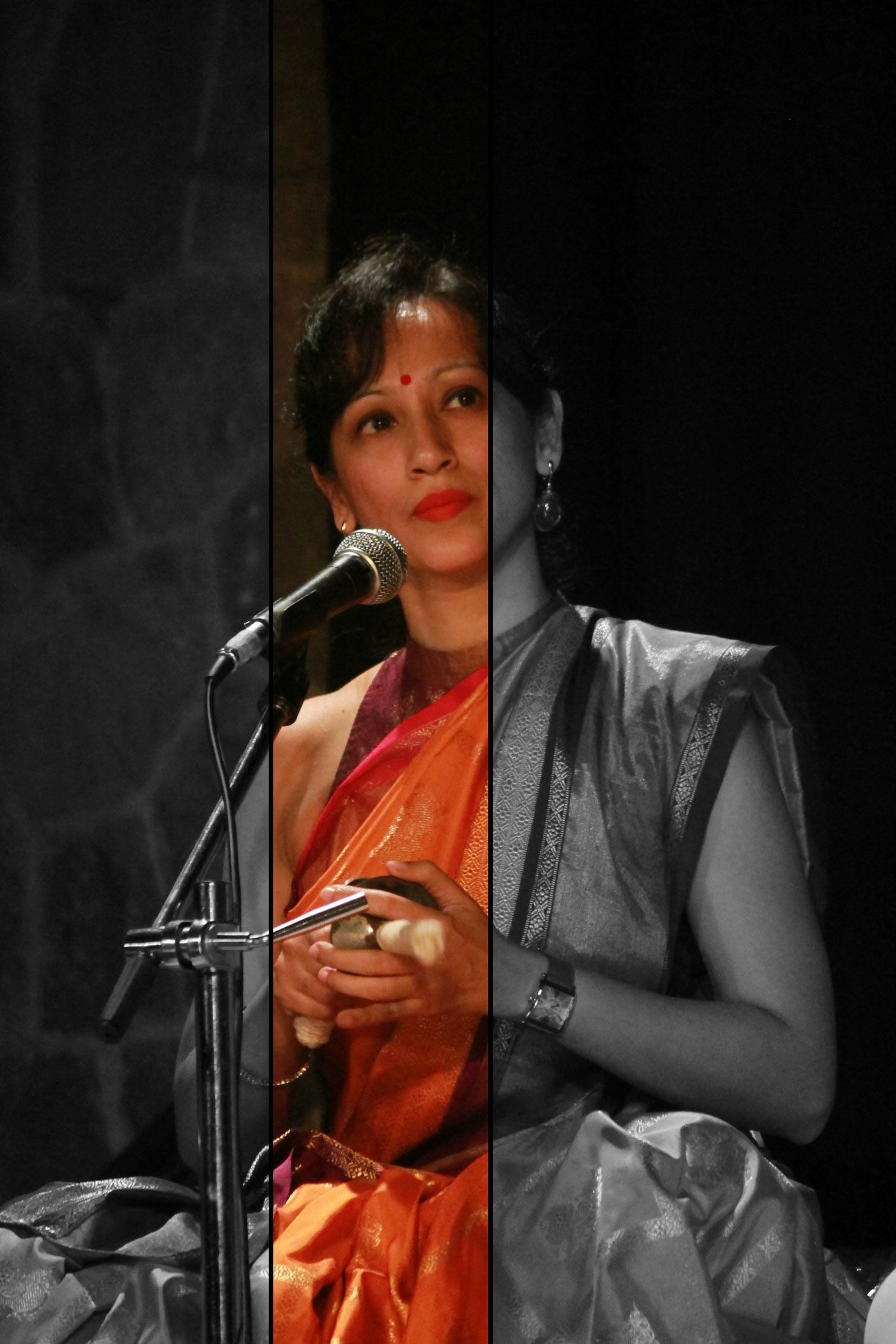# Blog

• HomeAnga of Rupaka Talam with Talalipi of all Jatis

The Rupaka Talam of the South Indian Tala System has only 2 angas- the Dhrutam which has 2 beats (symbolised by a 0) and a laghu which has variable beats according to the jati (symbolised by l).

Hence in talalipi, the symbol of Rupaka talam is 0l

If we have to write this talam in talalipi, the following possibilities can be created:
1. Tisra Jati Rupaka talam (where laghu will have only 3 beats- making a total of 5 beats in each avartanam). It can be written as :

S R l S R G ll
R G l R G M ll
G M l G M P ll
M P l M P D ll
P D l P D N ll
D N l D N S ll
S N l S N D ll
N D l N D P ll
D P l D P M ll
P M l P M G ll
M G l M G R ll
G R l G R S ll

2. Chatusra jati Rupaka talam (where laghu will have 4 beats making a total of 6 beats in each avartanam) can be written as :

S R l S R G M ll
R G l R G M P ll
G M l G M P D ll
M P l M P D N ll
P D l P D N S ll
S N l S N D P ll
N D l N D P M ll
D P l D P M G ll
P M l P M G R ll
M G l M G R S ll

3. Misra jati Rupaka talam (where the laghu has 7 beats making a total of 9 beats) can be written as :

S . l R . G . S R G ll
R . l G . M . R G Mll
G . l M . P . G M P ll
M . l P . D . M P D ll
P . l D . N . P D N ll
D . l N . S . D N S ll
S . l N . D . S N D ll
N . l D . P . N D P ll
D . l P . M . D P M ll
P . l M . G . P M G ll
M . l G . R . M G R ll
G . l R . S . G R S ll

4. Khanda jati Rupaka Talam (where the laghu has 5 beats making a total of 7 beats)can be written as follows:

S R l G S R G M ll
R G l M R G M P ll
G M l P G M P D ll
M P l D M P D N ll
P D l N P D N S ll
S N l D S N D P ll
N D l P N D P M ll
D P l M D P M G ll
P M l G P M G R ll
M G l R M G R S ll

5. Sangeerna jati Rupaka Talam (where the laghu has 9 beats creating a total of 11 beats) can be written as follows:

S R l G . S R G M . M . ll
R G l M . R G M P . P . ll
G M l P . G M P D . D . ll
M P l D . M P D N . N . ll
P D l N . P D N S . S . ll
S N l D . S N D P . P . ll
N D l P . N D P M . M . ll
D P l M . D P M G . G . ll
P M l G . P M G R . R . ll
M G l R . M G R S . S . ll